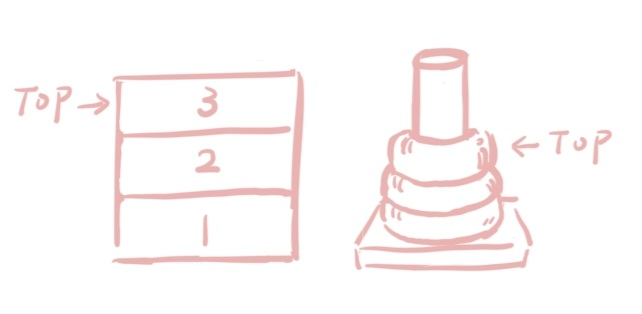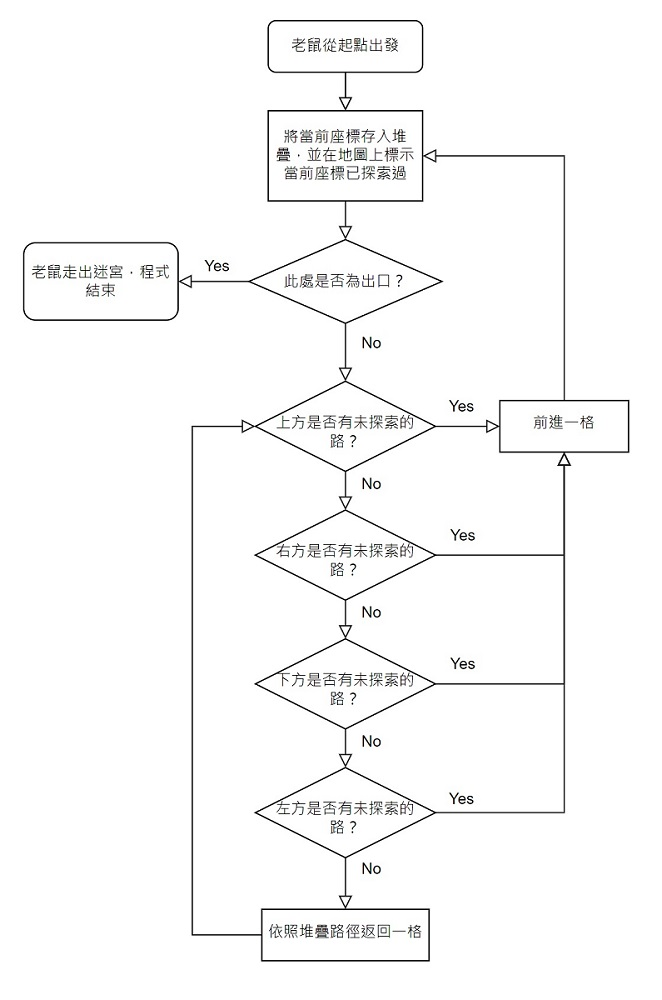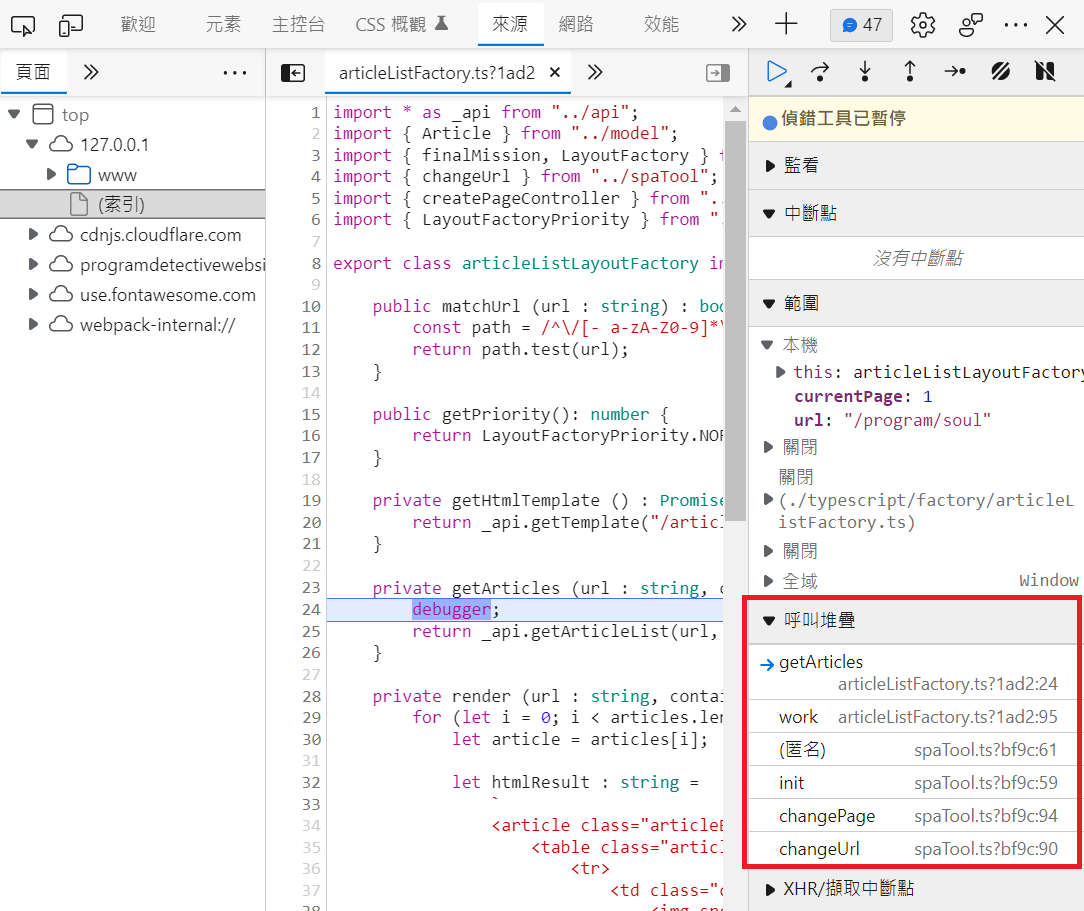# 堆疊(stack)是什麼？何時會用到這種資料結構？## 如何實作堆疊？

``````let stack = [];
let topIndex = -1;

// 存入(push)堆疊
stack[++topIndex] = "a";
stack[++topIndex] = "b";
stack[++topIndex] = "c";

// 從堆疊取出(pop)
let c = stack[topIndex--];
stack = stack.slice(0, stack.length - 1);
let b = stack[topIndex--];
stack = stack.slice(0, stack.length - 1);
let a = stack[topIndex--];
stack = stack.slice(0, stack.length - 1);``````

stack = stack.slice(0, stack.length - 1); 在這裡的意思是，把陣列裡最後一個元素移除，同時陣列的長度也會縮減，若最後 stack 的長度為 0，表示堆疊裡已經沒有東西了。

``````let stack = [];

// 存入(push)堆疊
stack.push("a");
stack.push("b");
stack.push("c");

// 從堆疊取出(pop)
let c = stack.pop();
let b = stack.pop();
let a = stack.pop();``````

push() 會從陣列前端開始存入資料，pop() 會從陣列尾端依序取出資料，執行結果會跟第一個例子一模一樣

## 經典例子

(1) 站在起點，把起點的座標丟入堆疊，並在地圖上標記這格為已探索。

(2) 檢查上方是否有未探索過的路，若有則前進一格，並把這格的座標丟入堆疊，另在地圖上標記為己探索。

(3) 如果上方不能走，就檢查右方是否有未探索過的路，若有則前進一格，並把這格的座標丟入堆疊，另在地圖上標記為已探索，然後再次從(2)開始判斷。

(4) 如果右方不能走，就檢查下方是否有未探索過的路，若有則前進一格，並把這格的座標丟入堆疊，另在地圖上標記為已探索，然後再次從(2)開始判斷。

(5) 如果下方不能走，就檢查左方是否有未探索過的路，若有則前進一格，並把這格的座標丟入堆疊，另在地圖上標記為已探索，然後再次從(2)開始判斷。

(6) 如果左方不能走，表示老鼠走到死路了，我們要依照堆疊的記錄沿途返回，每返回一格都要再次從(2)開始判斷。## 呼叫堆疊

``````1.  let a = 10;
2.  let b = 20;
3.  let result = 0;
4.
6.  console.log("結果: " + result);
7.
9.    return a + b;
10. }``````

（為求說明簡單，此處省略了程式計數器記憶體位址等較複雜的觀念，但概念上大致相同。）

## 總結` `

` `

X

email（選填，僅站長可見。）